### Home > CAAC > Chapter 5 > Lesson 5.1.5 > Problem5-50

5-50.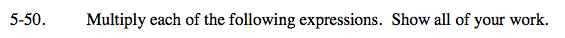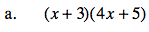Use a generic rectangle to multiply.

Put the products on the outside of the rectangle.

Use length times width to find the areas of the small rectangles.

Write the total area as a sum.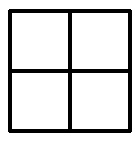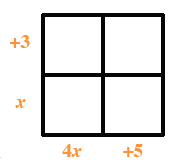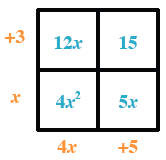4x2 + 12x + 5x + 15

4x2 + 17x + 15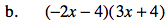Follow the steps in part (a).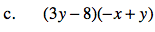Follow the steps in part (a).

−6x3 − 20x2 − 16x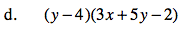Follow the steps in part (a).

3xy + 5y2 − 22y − 12x + 8